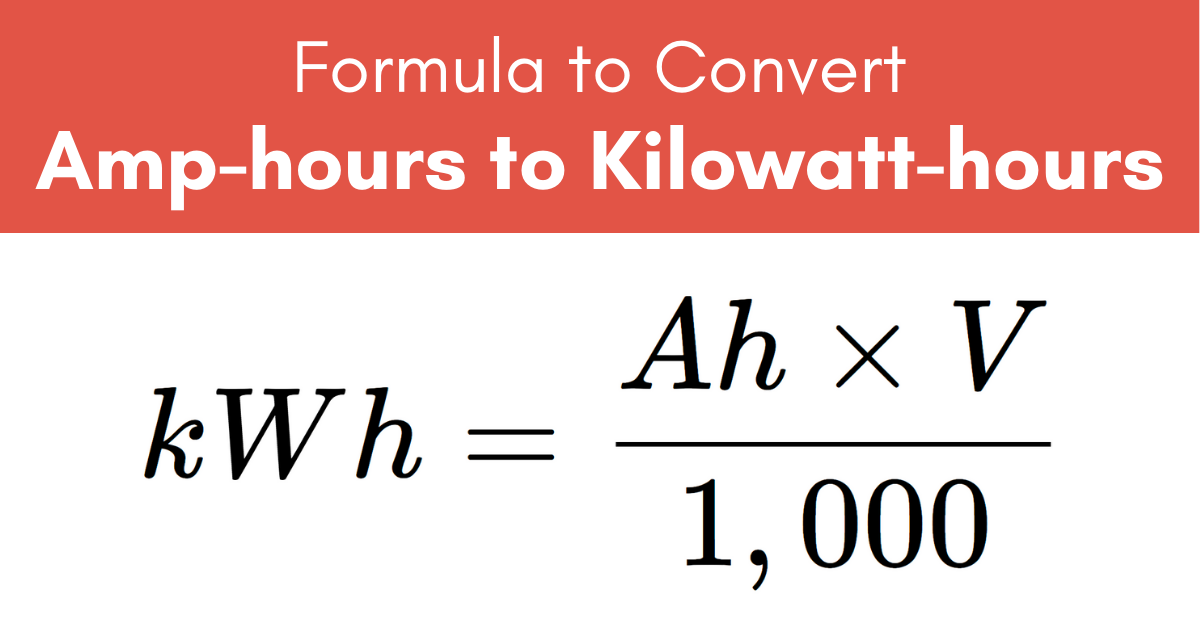# Amp-Hours (Ah) to Kilowatt-Hours (kWh) Conversion Calculator

Convert amp-hours to kilowatt-hours using the calculator below and entering the charge in Ah along with the voltage.

Ah
V

## Kilowatt-Hours Results:

kWh

Do you want to convert kWh to Ah instead?

## How to Convert Amp-Hours to Kilowatt-Hours

Amp-hours, often expressed as Ah or A·h, are a measure of electrical charge. Amp-hours are often used to measure the charge of a battery, for example. A charge of one Ah will supply one amp of current for one hour.

Kilowatt-hours, expressed kWh or kW·h, are used to measure electrical energy. One kWh is equal to one kilowatt, or one thousand watts, of energy consumed for one hour of time.

To convert from electrical charge to energy, use the formula below along with the voltage.

### Ah to kWh Conversion Formula

kWh = Ah × V1,000

The electrical energy in kilowatt-hours is equal to the charge in amp-hours times the voltage, then divided by 1,000.For example, let’s convert 20 Ah at 120 V to kWh.

kWh = (20 Ah × 120 V) ÷ 1,000
kWh = 2,400 ÷ 1,000
kWh = 2.4 kWh

You might be interested in our milliamp-hours to watt-hours calculator.